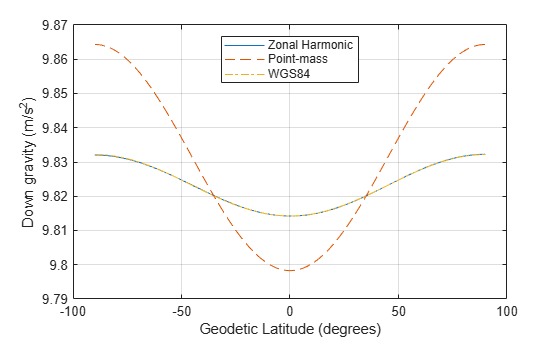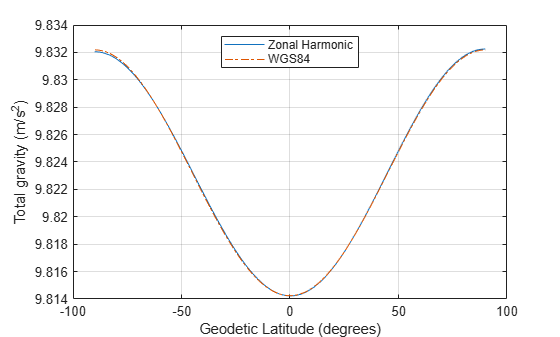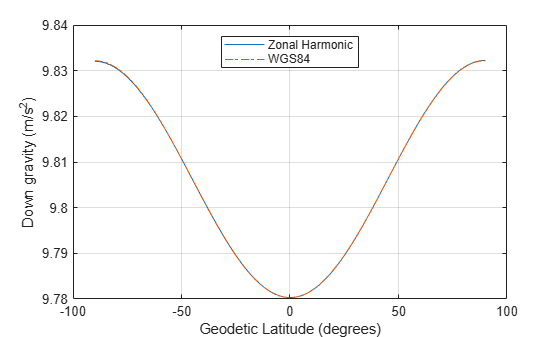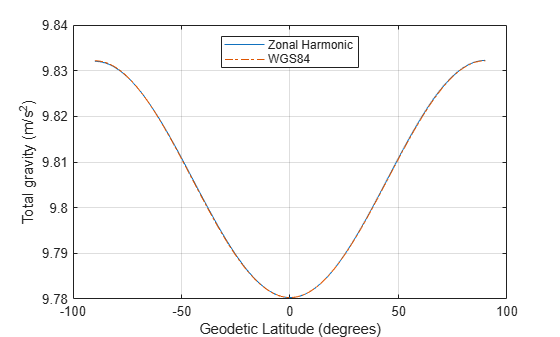# Comparing Zonal Harmonic Gravity Model to Other Gravity Models

This example shows how to examine the zonal harmonic, spherical, and 1984 World Geodetic System (WGS84) gravity models for latitudes from +/- 90 degrees at the surface of the Earth.

### Determine Earth-Centered Earth-Fixed (ECEF) Position

Since the ECEF coordinate system is geocentric, you can use spherical equations to determine the position from the geocentric latitude, longitude, and geocentric radius.

Calculate the geocentric radii in meters at an array of latitudes from +/- 90 degrees using `geocradius`.

```lat = -90:90; r = geocradius( lat ); rlat = convang( lat, 'deg', 'rad'); z = r.*sin(rlat);```

Because longitude has no effect for zonal harmonic gravity model, assume that the y position is zero.

```x = r.*cos(rlat); y = zeros(size(x));```

### Compute Zonal Harmonic Gravity for Earth

Use `gravityzonal` to calculate zonal harmonic gravity components in the ECEF coordinate system in meters per seconds squared for an array of ECEF position inputs.

`[gx_zonal, gy_zonal, gz_zonal] = gravityzonal([x' y' z']);`

### Calculate WGS84 Gravity

Use `gravitywgs84` to compute WGS84 gravity in down-axis and north-axis at the Earth's surface. WGS84 gravity is an array of geodetic latitudes in degrees and 0 degrees longitude computed using the exact method with atmosphere, no centrifugal effects, and no precessing.

```lat_gd = geoc2geod(lat, r); long_gd = zeros(size(lat)); [gd_wgs84, gn_wgs84] = gravitywgs84( zeros(size(lat)), lat_gd, long_gd, 'Exact', [false true false 0] );```

### Determine Gravity for Spherical Earth

Compute the array of point-mass gravity for the array of geocentric radii in meters per second squared using the Earth's gravitational parameter in meters cubed per second squared.

```GM = 3.986004415e14; gd_sphere = -GM./(r.*r);```

### Comparison Plots for Different Gravity Models

To compare the gravity models, their outputs must be in the same coordinate system. You can transform zonal gravity from ECEF coordinates to NED coordinates by using the Direction Cosine Matrix from `dcmecef2ned`.

```dcm_ef = dcmecef2ned( lat_gd, long_gd ); gxyz_zonal = reshape([gx_zonal gy_zonal gz_zonal]', [3 1 181]); gned_zonal = squeeze(pagemtimes(dcm_ef,gxyz_zonal))'; figure(1) plot(lat_gd, gned_zonal(:,3), lat, -gd_sphere, '--', lat_gd, gd_wgs84, '-.') legend('Zonal Harmonic', 'Point-mass', 'WGS84','Location','North') xlabel('Geodetic Latitude (degrees)') ylabel('Down gravity (m/s^2)') grid on```Figure 1: Gravity in the Down-axis in meters per second squared

```figure(2) plot( lat_gd, gned_zonal(:,1), [lat(1) lat(end)], [0 0], '--', lat_gd, gn_wgs84, '-.'); legend('Zonal Harmonic', 'Point-mass', 'WGS84','Location','Best') xlabel('Geodetic Latitude (degrees)') ylabel('North gravity (m/s^2)') grid on hold off```Figure 2: Gravity in the North-axis in meters per second squared

Calculate total gravity for WGS84 and from zonal gravity vector in meters per second squared.

```gtotal_wgs84 = gravitywgs84( zeros(size(lat)), lat_gd, zeros(size(lat)), 'Exact', [false true false 0] ); gtotal_zonal = sqrt(sum([gx_zonal.^2 gy_zonal.^2 gz_zonal.^2],2));```
```figure(3) plot( lat, gtotal_zonal, lat_gd, gtotal_wgs84, '-.' ) legend('Zonal Harmonic', 'WGS84','Location','North') xlabel('Geodetic Latitude (degrees)') ylabel('Total gravity (m/s^2)') grid on```Figure 3: Total gravity in meters per second squared

### Compare Gravity Models with Centrifugal Effects

Now, you have seen the gravity comparisons of a non-rotating Earth. Examine the centrifugal effects from the Earth's rotation on the gravity models.

### Compute Gravity Centrifugal Effects for Earth

Use `gravitycentrifugal` to calculate array of centrifugal effects in ECEF coordinates for array of ECEF positions in meters per seconds squared.

`[gx_cent, gy_cent, gz_cent] = gravitycentrifugal([x' y' z']);`

Add centrifugal effects to zonal harmonic gravity.

```gx_cent_zonal = gx_zonal + gx_cent; gy_cent_zonal = gy_zonal + gy_cent; gz_cent_zonal = gz_zonal + gz_cent;```

### Calculate WGS84 Gravity with Centrifugal Effects

Use `gravitywgs84` to compute WGS84 gravity in down-axis and north-axis at the Earth's surface. WGS84 gravity is an array of geodetic latitudes in degrees and 0 degrees longitude computed using the exact method with atmosphere, centrifugal effects, and no precessing.

`[gd_cent_wgs84, gn_cent_wgs84] = gravitywgs84( zeros(size(lat)), lat_gd, long_gd, 'Exact', [false false false 0] );`

Calculate total gravity with centrifugal effects for WGS84 and from zonal gravity vector in meters per second squared.

```gtotal_cent_wgs84 = gravitywgs84( zeros(size(lat)), lat_gd, zeros(size(lat)), 'Exact', [false false false 0] ); gtotal_cent_zonal = sqrt(sum([gx_cent_zonal.^2 gy_cent_zonal.^2 gz_cent_zonal.^2],2));```

### Comparison Plots for Different Gravity Models with Centrifugal Effects

To compare the gravity models, their outputs must be in the same coordinate system. You can transform zonal gravity from ECEF coordinates to NED coordinates by using the Direction Cosine Matrix from `dcmecef2ned`. In figure 5, you can see there is some difference between zonal harmonic gravity with centrifugal effects and WGS84 gravity with centrifugal effects. The majority of difference is due to differences between the zonal harmonic gravity and WGS84 gravity calculations.

```gxyz_cent_zonal = reshape([gx_cent_zonal gy_cent_zonal gz_cent_zonal]', [3 1 181]); gned_cent_zonal = squeeze(pagemtimes(dcm_ef,gxyz_cent_zonal))'; figure(4) plot(lat_gd, gned_cent_zonal(:,3), lat_gd, gd_cent_wgs84, '-.') legend('Zonal Harmonic', 'WGS84','Location','North') xlabel('Geodetic Latitude (degrees)') ylabel('Down gravity (m/s^2)') grid on```Figure 4: Gravity with centrifugal effects in the Down-axis in meters per second squared

```figure(5) plot( lat_gd, gned_cent_zonal(:,1), lat_gd, gn_cent_wgs84, '--', lat_gd, (gned_zonal(:,1)-gn_wgs84'), '-.' ) axis([-100 100 -0.0002 0.0002]) legend('Zonal Harmonic', 'WGS84', 'Error Between Models w/o Centrifugal Effects', 'Location','Best') xlabel('Geodetic Latitude (degrees)') ylabel('North gravity (m/s^2)') grid on```Figure 5: Gravity in the North-axis in meters per second squared

```figure(6) plot( lat, gtotal_cent_zonal, lat_gd, gtotal_cent_wgs84, '-.' ) legend('Zonal Harmonic', 'WGS84','Location','North') xlabel('Geodetic Latitude (degrees)') ylabel('Total gravity (m/s^2)') grid on```Figure 6: Total gravity with centrifugal effects in meters per second squared